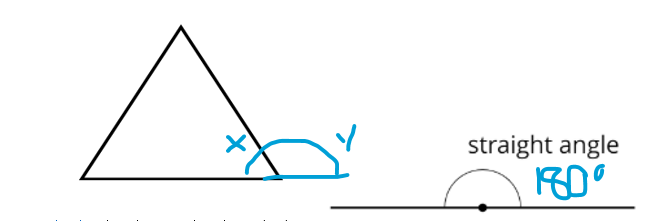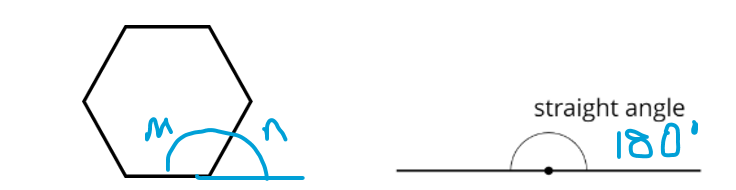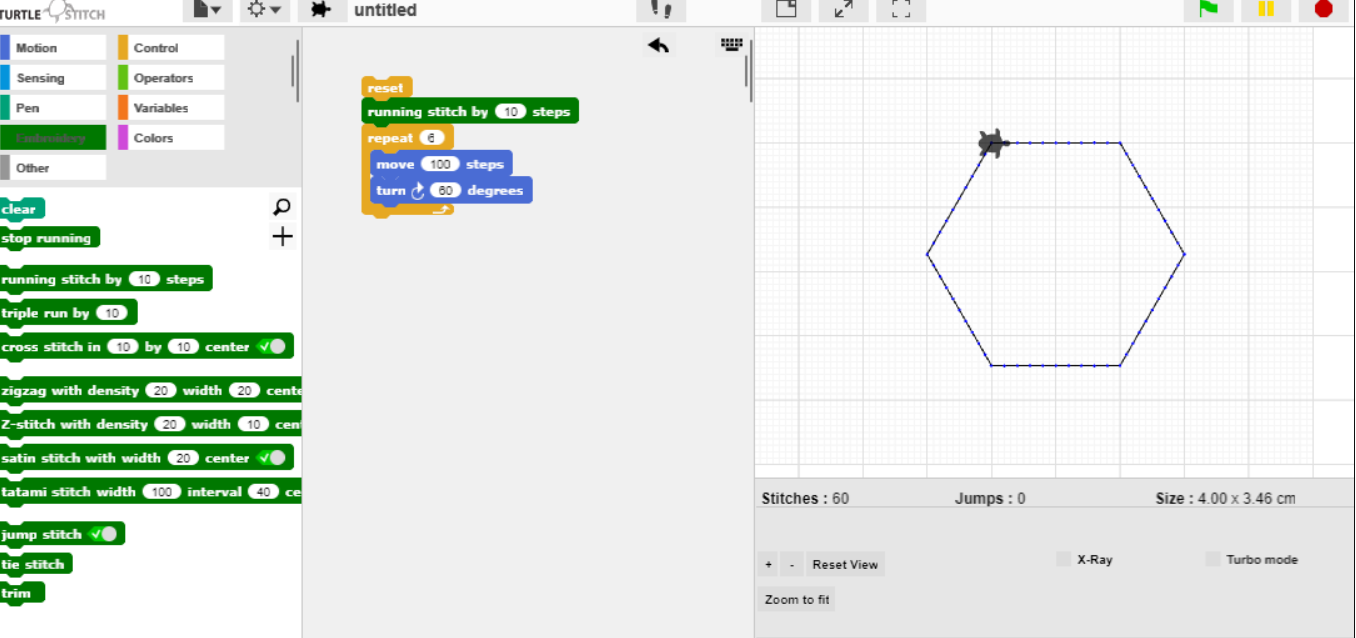# TurtleStitch

### Finding Angles

When you are creating a pattern on TurtleStitch, there is a key element into getting your pattern right and that is the number of degrees your turtle must turn.

Here, we will look at a few examples of how to find missing angles.

The first shape I will look at will be a Triangle.1. This is an equilateral triangle, all the interiors of triangles equal 180 degrees. An equilateral triangle means it has three equal sides. This means we divide 180 by 3, to get our 3 inside angles. 180 divided 3 is 60.

2. Now take 60 from a 180. This equals 120 degrees.

3. This means that x = 60 and y equals 120. So your turn degrees in your block of code will be set to 120. This gives us our 60 degree angles in our triangle.

The next shape we will be looking at is a regular hexagon.1. A hexagon has 6 sides, the interior equals 720. You need to divide 720 by 6. This gives you a result of 120.

2. Take 120 from 180 and you will be given the result of 60.

3. This means n = 60 degrees and m = 120 degrees.

4. Your turn degrees will be 60 which then gives you the perfect angles inside of your hexagon of 120. Remember that a hexagon has 6 sides so your repeat loop will need to be 6 instead of 10.

See images below of code and pattern:Created by: Hope Boyle